Home

ARTICLE 50 (Part 2)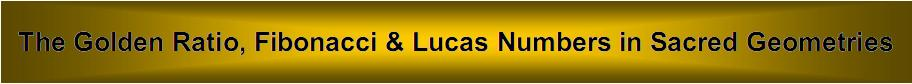by

Stephen M. Phillips

Flat 4, Oakwood House, 117-119 West Hill Road. Bournemouth. Dorset BH2 5PH. England.

Website: http://smphillips.mysite.com

Abstract

 The polyhedral form of the Tree of Life is the 144 Polyhedron with 144 faces and the disdyakis triacontahedron with 120 faces. They are symbolized by the 144 yods inside the boundaries of the seven, tetractys-constructed, enfolded polygons of the inner Tree of Life, which are lined by 120 yods. The 74 vertices of the 144 Polyhedron correspond to the 74 yods up to the top of the lowest Tree of Life that are not Sephiroth. The 62 vertices of the disdyakis triacontahedron correspond to the 62 corners of the inner Tree of Life that are unshared with its outer form. The faces of the rhombic dodecahedron that generate the 144 polyhedron are rhombi with the proportions of √2:1. The faces of the rhombic triacontahedron that underlies the disdyakis triacontahedron are Golden Rhombi with the proportions of the Golden Ratio. Both types of rhombi are implicit in the geometry of the outer Tree of Life. The Golden Rhombus is implicit in the Fano plane, which represents the algebra of the octonions. The 60 vertices surrounding an axis passing through two opposite C vertices lie in 15 sheets. They form 15 polygons which, together with the two C vertices, have 550 geometrical elements, that is, 10F10 , where F10 is the tenth Fibonacci number. The 299 elements in one half of the polyhedron and the remaining 251 elements are the counterpart of the 299 Sephiroth in the 49-tree that maps the cosmic physical plane and the 251 Sephiroth in the 42 Trees above it that map the six cosmic superphysical planes of consciousness. The 550 geometrical elements (370 vertices & sides, 180 triangles) are the counterpart of the 550 geometrical elements (370 vertices & sides, 180 triangles) in the 50 faces of the five Platonic solids. The 34 (F9) corners of the 27 sectors in each set of seven polygons either above or below the central one correspond to the 34 corners associated with each set of seven enfolded polygons that are unshared with polygons enfolded in the next higher Tree. The 34 corners of the 21 triangles in each half of the two-dimensional Sri Yantra are their counterpart. The ninth Lucas number 76 is the number of vertices & centres of polygons that surround the centre of the disdyakis triacontahedron. The tenth Lucas number 123 is the number of points & lines shaping the 15 polygons. Equivalent to the I Ching table of 64 hexagrams and the 7-tree, the Sri Yantra embodies the superstring structural parameter 1680. This is also embodied in the 21 copies of the first four Platonic solids that fit the disdyakis triacontahedron. The 28 polyhedra that fit it embody the superstring structural parameter 3360. This is the number of yods in the seven enfolded polygons when their 47 sectors are 2nd-order tetractyses. F9 is the number of tree levels in the 10-tree, whose 361 sectors of 127 triangles have 550 sides. Prescribed by ADONAI, the Godname of Malkuth, the 1680 yods below its top when the sectors of their triangles are tetractyses symbolize the 1680 turns in each of the ten helical, closed curves of the E8×E8 heterotic superstring.

Page Index

 Part 1 Part 2 Page Page Table of number values of the Sephiroth in the four Worlds Table of number values of the Sephiroth in the four Worlds The Tree of Life Geometrical properties of the 13 Archimedean & 13 Catalan solids The Upper & Lower Faces of the Tree of Life The polygonal Tree of Life encodes its polyhedral counterpart Fibonacci & Lucas numbers Construction of the polyhedral Tree of Life Fibonacci & Lucas numbers in the 1-, 2- & 3-tree Isomorphism between the polygonal & polyhedral Trees of Life The generation of the inner Tree of Life √2 & Φ determine the polyhedral Tree of Life The outer & inner Tree of Life Connection between the Tree of Life and the faces of the rhombic dodecahedron & rhombic triacontahedron Fibonacci numbers in the geometry of the inner Tree of Life The Golden Rhombus in the Fano plane Geometrical composition of the outer & inner Tree of Life The 15 sheets of vertices in the disdyakis triacontahedron The ninth Fibonacci number in the outer & inner Tree of Life The disdyakis triacontahedron as the polyhedral representation of CTOL Fibonacci numbers & the Golden Ratio in the DNA molecule The 34 corners of the 27 sectors of the seven polygons Comparison of the 64 codons & 64 anticodons with the 64 hexagrams The two sets of 7 polygons are analogous to the two halves of the inner Tree of Life and the trunk and branches of the outer Tree of Life Comparison of the 384 geometrical elements of the 14 polygons with the 384 lines & broken lines in the 64 hexagrams The ninth & tenth Lucas numbers determine the 15 sheets of vertices Comparison of the 64 hexagrams with the Sri Yantra The disdyakis triacontahedron embodies the fine-structure number 137 The five Platonic solids as a sequence governed by Fibonacci numbers The equivalence of the outer Tree of Life and the Sri Yantra F8, F9 & F10 in the five Platonic solids The equivalence of the inner Tree of Life and the Sri Yantra The first four Platonic solids and the square embody the dimension 248 of E8 The equivalence of the Sri Yantra and 7 overlapping Trees of Life How the 1-tree embodies the dimension 248 of E8 The equivalence of the Sri Yantra and the 7-tree How the (7+7) separate polygons of the inner Tree of Life embody E8×E8 The Sri Yantra embodies the superstring structural parameter 1680 1370 yods on edges of tetractyses in the five Platonic solids Numbers of hexagonal yods in the faces of the Platonic solids The inner Tree of Life contains 1370 yods Correspondence between the outer and inner Trees of Life Fibonacci, Lucas numbers and the Golden Ratio determine the geometrical composition of the five Platonic solids 28 polyhedra fit into the disdyakis triacontahedron The number 247 is a Tree of Life parameter The faces of the 28 polyhedra have 3360 hexagonal yods Properties of the five Platonic solids The seven enfolded polygons of the inner Tree of Life have 3360 yods Counterpart of the internal geometrical composition of the Platonic solids in the inner Tree of Life EHYEH prescribes the superstring structural parameter 1680 Counterpart of the internal composition of the Platonic solids in the inner form of ten Trees of Life 49 overlapping Trees of Life contain 1680 geometrical elements The 3-tree determined by the tenth Lucas number encodes the human skeleton 1680 geometrical elements surround an axis of the disdyakis triacontahedron The 3-tree encodes the 361 acupuncture points The number value of Cholem Yesodeth is 168 The eight Church musical modes Geometrical composition of the Archimedean & Catalan solids Tone ratios of the notes in the seven octave species The Godname ADONAI prescribes the 10-tree The tenth Lucas number is the number of Pythagorean intervals between notes of the seven musical scales 1680 yods lie below the top of the 10-tree References The 10-tree has 34 tree levels References

1

 SEPHIRAH GODNAME ARCHANGEL ORDER OF ANGELS MUNDANE CHAKRA 1 Kether (Crown)                  620 EHYEH (I am)                  21 Metatron (Angel of the Presence)                  314 Chaioth ha Qadesh (Holy Living Creatures)                  833 Rashith ha Gilgalim First Swirlings. (Primum Mobile)                  636 2 Chokmah (Wisdom)                  73 YAHWEH, YAH (The Lord)                  26, 15 Raziel (Herald of the Deity)                  248 Auphanim (Wheels)                  187 Masloth (The Sphere of the Zodiac)                  140 3 Binah (Understanding)                  67 ELOHIM (God in multiplicity)                  50 Tzaphkiel (Contemplation of God)                  311 Aralim (Thrones)                  282 Shabathai Rest. (Saturn)                  317 Daath (Knowledge)                  474 4 Chesed (Mercy)                  72 EL (God)                  31 Tzadkiel (Benevolence of God)                  62 Chasmalim (Shining Ones)                  428 Tzadekh Righteousness. (Jupiter)                  194 5 Geburah (Severity)                  216 ELOHA (The Almighty)                  36 Samael (Severity of God)                  131 Seraphim (Fiery Serpents)                  630 Madim Vehement Strength. (Mars)                  95 6 Tiphareth (Beauty)                  1081 YAHWEH ELOHIM (God the Creator)                  76 Michael (Like unto God)                  101 Malachim (Kings)                  140 Shemesh The Solar Light. (Sun)                  640 7 Netzach (Victory)                  148 YAHWEH SABAOTH (Lord of Hosts)                  129 Haniel (Grace of God)                  97 Tarshishim or Elohim                  1260 Nogah Glittering Splendour. (Venus)                  64 8 Hod (Glory)                  15 ELOHIM SABAOTH (God of Hosts)                  153 Raphael (Divine Physician)                  311 Beni Elohim (Sons of God)                  112 Kokab The Stellar Light. (Mercury)                  48 9 Yesod (Foundation)                  80 SHADDAI EL CHAI (Almighty Living God)                  49, 363 Gabriel (Strong Man of God)                  246 Cherubim (The Strong)                  272 Levanah The Lunar Flame. (Moon)                  87 10 Malkuth (Kingdom)                  496 ADONAI MELEKH (The Lord and King)                  65, 155 Sandalphon (Manifest Messiah)                  280 Ashim (Souls of Fire)                  351 Cholem Yesodeth The Breaker of the Foundations. The Elements. (Earth)                  168

 The Sephiroth exist in the four Worlds of Atziluth, Beriah, Yetzirah and Assiyah. Corresponding to them are the Godnames, Archangels, Order of Angels and Mundane Chakras (their physical manifestation). This table gives their number values obtained by the ancient practice of gematria, wherein a number is assigned to each letter of the alphabet, thereby giving a number value to a word that is the sum of the numbers associated with its letters. When some of these numbers are referred to in the article, they will be written in boldface.

 Figure 2 There are 13 types of semi-regular polyhedra whose faces are two or more types of regular polygons. They are called the “Archimedean solids.” Two are chiral, having partners that are mirror images of them. The dual of a polyhedron with C vertices and F faces is one with F vertices and C faces. The duals of the Archimedean solids are the Catalan solids. Figure 2 displays the numbers of vertices (C), edges (E) & faces (F) in the 30 Archimedean and Catalan solids, including their chiral versions. They are listed in order of increasing numbers of vertices for the Archimedean solids and increasing numbers of faces for the Catalan solids. The last of the latter in the list is the disdyakis triacontahedron, which has 62 vertices, 180 edges & 120 faces. 62 is the number value of Tzadkiel, the Archangel of Chesed.

2

 F E C Archimedean solid 8 18 12 truncated tetrahedron 14 24 12 cuboctahedron 14 36 24 truncated cube 14 36 24 truncated octahedron 26 48 24 rhombicuboctahedron 38 60 24 snub cube 38 60 24 snub cube (chiral partner) 32 60 30 icosidodecahedron 26 72 48 truncated cuboctahedron 32 90 60 truncated icosahedron 32 90 60 truncated dodecahedron 62 120 60 rhombicosidodecahedron 92 150 60 snub dodecahedron 92 150 60 snub dodecahedron (chiral partner) 62 180 120 truncated icosidodecahedron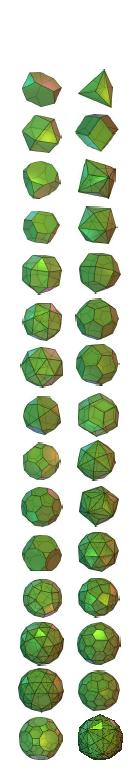Catalan solid F E C triakis tetrahedron 12 18 8 rhombic dodecahedron 12 24 14 triakis octahedron 24 36 14 tetrakis hexahedron 24 36 14 deltoidal icositetrahedron 24 48 26 pentagonal icositetrahedron 24 60 38 pentagonal icositetrahedron (chiral partner) 24 60 38 rhombic triacontahedron 30 60 32 disdyakis dodecahedron 48 72 26 triakis icosahedron 60 90 32 pentakis dodecahedron 60 90 32 deltoidal hexacontahedron 60 120 62 pentagonal hexacontahedron 60 150 92 pentagonal hexacontahedron (chiral partner) 60 150 92 disdyakis triacontahedron 120 180 62

Figure 3

With their 47 sectors turned into tetractyses, the seven enfolded polygons making up half of the inner Tree of Life have 264 yods. 120 yods line their boundaries, where

120 = 22 + 42 + 62 + 82,

and 144 yods are inside their sides, where

 144 = 10   20  30   40 11   21  31  41 12   22  32  42 13   23  33  43.

Suppose that two polyhedral counterparts of the inner Tree of Life exist and that these two sets of yods symbolise their vertices or faces. According to Fig. 2, there are no two solids with 144 and 120 vertices. The truncated icosidodecahedron has 120 vertices, but none of these polyhedra has 144 vertices. Nor can such a polyhedron be created by sticking tetrahedra or pyramids onto faces of any Archimedean or Catalan solid. For that to be possible, C + F = 144, but none of them has this property. However, the disdyakis triacontahedron has 120 faces and sticking tetrahedra onto the 48 faces of the disdyakis dodecahedron generates a polyhedron with 144 faces. This allows the 120 yods on edges of the seven enfolded polygons to be interpreted as denoting the 120 faces of the last Catalan solid and the 144 internal yods as symbolizing the 144 faces of a polyhedron (hereafter called the “144 Polyhedron”).

3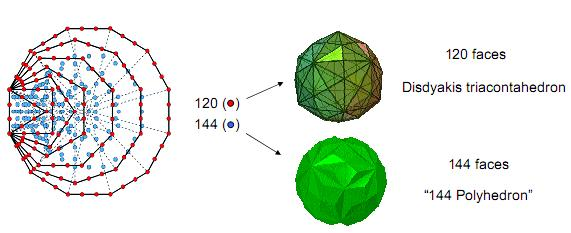The 120 red yods on the boundaries of the seven enfolded polygons symbolize the 120 faces of the disdyakis triacontahedron with 62 vertices. Their 144 internal, blue yods symbolize the 144 faces of a polyhedron with 74 vertices.

 Figure 4 The Catalan solid called the disdyakis dodecahedron has 26 vertices, 72 edges & 48 faces. Attaching tetrahedra to each face generates the 144 Polyhedron with (26+48=74) vertices, (72+3×48=216) edges and (3×48=144) triangular faces. 144 is F12, the twelfth Fibonacci number. 72 is the number value of Chesed, the first Sephirah of Construction and 216 is the number value of Geburah, the second Sephirah of Construction opposite it in the Tree of Life. The Catalan solid called the rhombic triacontahedron has 32 vertices, 60 edges & 30 so-called “Golden Rhombic” faces (to be described in Fig. 6). Attaching four-faced pyramids with Golden Rhombic bases to their faces generates the disdyakis triacontahedron with (32+30) vertices, (60+4×30=180) edges and (4×30=120) triangular faces. The 144 Polyhedron and the disdyakis triacontahedron constitute the polyhedral form of the Tree of Life. They represent the union of, respectively, the Yang (male) and Yin (female) aspects of Divine Unity.

 Figure 5 Imagine a set of overlapping Trees of Life with their triangles turned into tetractyses. Of the 84 yods up to the level of the top of the lowest Tree, ten are Sephiroth of that Tree, so that 74 more yods are needed to complete it. There are 26 yods down to the level of Daath, leaving 48 yods. 26 is the number value of YAHWEH, the Godname of Chokmah and 48 is the number value of Kokab, the Mundane Chakra of Hod. The 74 yods other than Sephiroth symbolise the 74 vertices of the 144 Polyhedron, the 26 yods denoting the 26 vertices of the underlying disdyakis dodecahedron and the 48 yods denoting the 48 vertices added by attaching tetrahedra to its 48 faces. When this Tree is projected onto the plane of the 14 polygons of the inner Tree of Life, seven Sephiroth and Daath coincide with some of the 70 corners of the polygons, leaving 62 corners that are intrinsic to them, being unshared with the projected outer Tree of Life. They denote the 62 vertices of the disdyakis triacontahedron. The 144 Polyhedron is the polyhedral version of the outer Tree of Life and the disdyakis triacontahedron is the polyhedral form of the inner Tree of Life. The 30 peaks of the Golden Rhombic pyramids in the disdyakis triacontahedron (called “A” vertices) correspond to the 30 yellow corners of the pentagons, hexagons & dodecagons. The 12 B vertices (vertices of an icosahedron) correspond to the 12 blue corners of the two octagons. The 20 C vertices (vertices of a dodecahedron) correspond to the 20 red corners of the squares & decagons.

5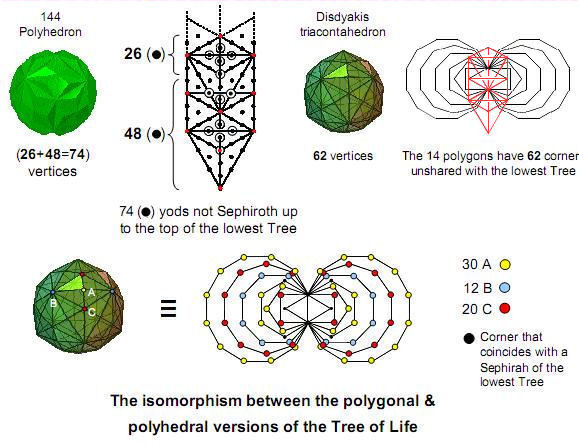The 12 faces of a rhombic dodecahedron are rhombi whose diagonals have lengths in the proportion of √2:1. Attaching rhombic pyramids to their faces creates the disdyakis dodecahedron with 48 faces. Attaching tetrahedra to the latter generates the 144 Polyhedron with 144 faces. 144 is the twelfth Fibonacci number. It is also 122. The geometrical meaning of this is that each of the 12 faces of the rhombic dodecahedron generates 12 faces of the 144 Polyhedron.   The rhombic triacontahedron has the 32 vertices of the underlying dodecahedron and icosahedron. The diagonals of its faces are their edges, the shorter (red) one being the edge of the former and the longer (green) one being the edge of the latter. The ratio of their lengths is the Golden Ratio Φ. Sticking Golden Rhombic pyramids onto the 30 faces of the rhombic triacontahedron creates the disdyakis triacontahedron. Whereas the Golden Ratio is only implicit in the geometry of the pentagonal faces of the dodecahedron, it becomes explicit in the proportions of the diamond-shaped bases of the 30 pyramids. The ideal of proportion is realised in the polyhedral Tree of Life.

6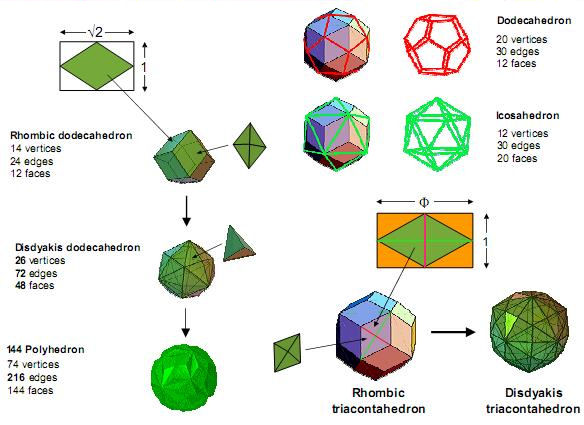The longer diagonal of the rhombic face of the rhombic dodecahedron is the edge of a cube. Its shorter diagonal is the edge of an octahedron. The ratio of their lengths is √2 = 1.414… . These Platonic solids are dual to each other. The two other Platonic solids that are dual to each other — the icosahedron and the dodecahedron — share an analogous property in that their edges are, respectively, the longer and shorter diagonals of the rhombic faces of the rhombic triacontahedron. Their ratio is the Golden Ratio Φ  = 1.618… . These rhombi are generated in a simple way by the geometry of the outer Tree of Life. Fig. 7 shows that the ten Sephiroth are the centres or points of intersection of a column of white circles. Let us take their radii as one unit. The central Pillar of Equilibrium intersects the path joining Chesed and Geburah at a point A that is one unit away from the vertical right-hand tangent BC to these circles. ABCD is a square with sides of length 1. Therefore, its diagonal AC = √(12 + 12)   = √2 . With A as centre, draw a circle passing through C of radius √2 (shown as a dashed line). It intersects the line drawn along AB at E. E′ i s the corresponding point on the other side of the central pillar. EE' = 2√2. The central pillar intersects the path joining Netzach and Geburah at D'. DD' = 2. Therefore, EE'/DD' = 2√2/2 = √2. We find that the rhombus DED'E' has the same shape as the rhombic face of the rhombic dodecahedron. Extend the tangent at B to the point F, where BC = CF = 1. Then, BF = 2 and AF = √( 22 + 12) = √5. The line AF intersects CD at G, where AG = GF = √5/2. With G as centre, draw a circle of radius ½. It intersects AF at H, where AH = √5/2 + ½ = (√5 +1)/2. This is Φ, the Golden Ratio. With A as centre, draw a circle of radius AH. It intersects the extension of AB at I, where AI = Φ.  I' is its counterpart on the other side of the central pillar. II' = 2Φ. Therefore, II'/DD' = 2Φ/2 = Φ. The rhombus DI D'I' has the same shape as the rhombic face of the rhombic triacontahedron. What manifests finally as the fruit of the Tree of Life, namely, the disdyakis triacontahedron with golden rhombic faces, was within it as their seed shape from the very beginning!

7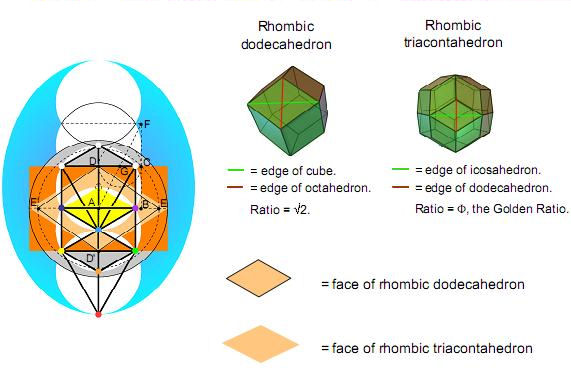The geometry of the Tree of Life generates the rhombic faces of the rhombic dodecahedron & rhombic triacontahedron.

 The Fano plane is known to mathematicians as the simplest projective plane. It has seven points and seven lines (three sides and three internal diagonals of a triangle and the circle inscribed in it). The Golden Rhombus is implicit in its geometry. The Fano plane, which represents the algebra of the octonions, has the 168 symmetries of the symmetry group SL(3,2), which is the symmetry group of G2, the rank-2 exceptional group. This is the symmetry group of the octonions. SL(3,2) is isomorphic to PSL(2,7), the symmetry group of the Klein quartic:   X3Y + Y3Z + Z3X = 0.   Given that its dimension is 168 , can it be just coincidental that the disdyakis triacontahedron, whose groups of four faces have the outline of a Golden Rhombus, have 1680 vertices, lines & triangles surrounding an axis joining two opposite vertices when its interior triangles are divided into their sectors (1)? This number is the number of circularly polarised oscillations in each of the ten closed curves making up the E8×E8 heterotic superstring, as described by the Theosophists Annie Besant & C.W. Leadbeater over a century ago (2). It prove that the helical structure of the basic unit of matter is embodied in the geometrical composition of the polyhedral Tree of Life blueprint.

 Consider a straight line passing through two opposite C vertices of the disdyakis triacontahedron. The 60 vertices surrounding this axis are grouped into 15 sheets. Seven sheets (coloured with the seven colours of the rainbow) are above the central one (coloured khaki) and seven sheets are below it. Projected onto the plane containing the central sheet, the vertices form triangles and six-sided polygons that are irregular except for the central one, which is hexagonal. The rectangular coordinates of the 62 vertices are arranged according their height (Z' coordinate) measured along the axis. The vertices in a sheet of a given colour have the same height. The numbers between the arrows indicate the numbers of vertices in each sheet. Vertices 4 and 59 in the white rows are the diametrically opposite C vertices through which the axis passes. The pattern of 31 vertices below the thick black line is the mirror image of the pattern of 31 vertices above it. 31 is the number value of EL, the Godname of Chesed. 62 is the number value of Tzadkiel , its Archangel. For more details see Article 36 (3).

9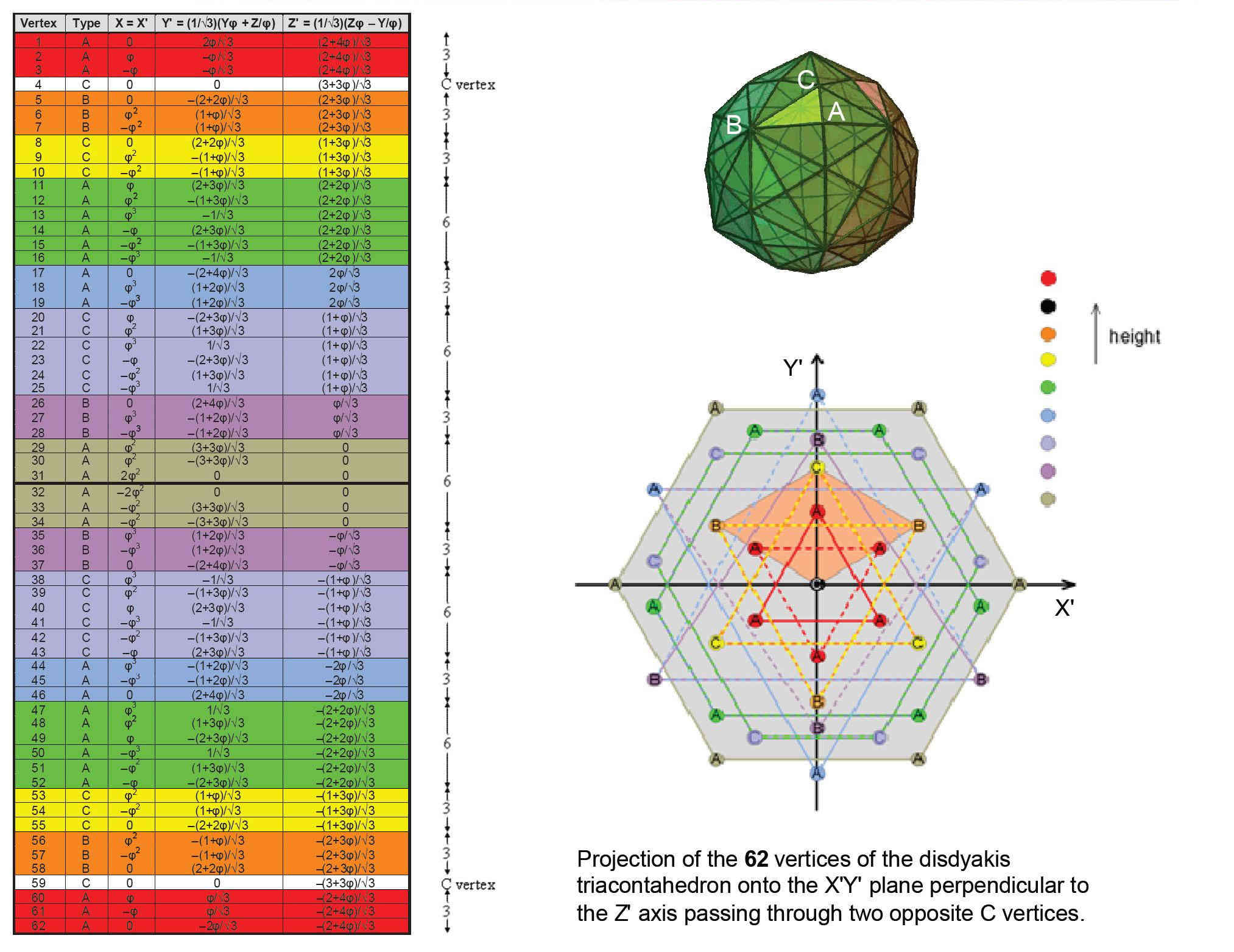Sacred geometries are maps of all levels of physical and superphysical realities (4). In particular, their Tree of Life representation is called the "Cosmic Tree of Life" (CTOL). It consists of 91 overlapping Trees of Life with 550 Sephirothic levels (SLs), where 550 = 10×55 and 55 is the tenth Fibonacci number. We saw in Part 1 that this is the number of corners, sides and triangles in the 50 faces of the five Platonic solids (see Fig. 16 and its commentary). There are 299 SLs up to the top of the lowest 49 Trees of Life ("49-tree”) and 251 SLs above it. The 49-tree maps the 49 subplanes of the seven planes of consciousness taught in Theosophy. Above it are the 42 Trees of Life mapping the 42 subplanes of the six cosmic superphysical planes.   Suppose that the 15 polygons formed by the 60 vertices surrounding an axis passing through two opposite C vertices are divided into their sectors. Then suppose that each of the 60 sectors is again divided into its three sectors. Inspection of the projection of vertices onto the central sheet shows that none of the sides of the polygons is an edge of the disdyakis triacontahedron. If one imagines all the vertices joined to the centre so as to form triangles with polyhedral edges as one side, the central hexagon is the only polygon whose internal sides of sectors coincide with these triangles. They are coloured red in Fig. 10. The two C vertices on the axis are unshared with the 15 polygons. Listed in the table are the numbers of points, lines & triangles making up the polygons (including these two vertices) that are unshared (the centre of the central hexagon is shared with internal triangles of the polyhedron and so is not included in the numbers). The lower half of the disdyakis triacontahedron has eight polygons with 299 unshared geometrical elements (including the lower C vertex). They correspond to the 299 SLs in the 49-tree. The seven polygons and the upper C vertex comprise 251 geometrical elements. They correspond to the 251 SLs above the 49-tree. CTOL is encoded in the geometry of the polygons formed by the vertices of the disdyakis triacontahedron. To every one of the 550 geometrical elements there corresponds an SL.  There are 76 points (60 points surrounding the axis, 14 centres & two C vertices). This is the ninth Lucas number L9. 76 is the number value of YAHWEH ELOHIM, the Godname of Tiphareth. There are 370 unshared vertices & sides of 180 triangles. Compare this with the 370 vertices & sides of the 180 triangles in the faces of the five Platonic solids (see Fig. 16). The holistic parameter 550 divides in exactly the same way! There are 474 sides & triangles. 474 is the number value of Daath (“knowledge”). Truly, the number 550 measures the knowledge of YAHWEH ELOHIM (“God the Creator”), being the number of SLs in CTOL.

 The seven polygons above or below the central sheet of vertices in the disdyakis triacontahedron have 27 sectors with 34 corners. 34 is the ninth Fibonacci number F9. Dividing each sector into three triangles and adding the C vertex associated with them generates 55 vertices other than centres of polygons, where 55 is the tenth Fibonacci number F10.   The two sets of seven polygons above and below the central sheet have 54 sectors with (2×34 =68) corners. This is the counterpart of the 68 corners per set of (7+7) polygons enfolded in each overlapping Tree of Life, 34 corners per set of seven polygons (see Fig. 10, Part 1). This property serves to illustrate that the disdyakis triacontahedron is the polyhedral counterpart of the inner Tree of Life, as has been shown in many previous articles.

11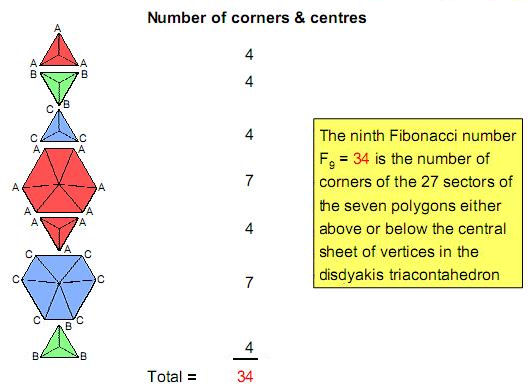Another counterpart of the disdyakis triacontahedron to the inner Tree of Life is as follows: associated with each set of seven enfolded polygons are their 35 corners (34 outside their shared root edge and one corner that is an endpoint of the latter). The 27 sectors of each set of seven polygons in the disdyakis triacontahedron have 34 corners. Including the single C vertex on the axis that is associated with each set, there are 70 vertices and centres surrounding the central polygon, 35 per set of seven polygons & C vertex). The trunk of the outer Tree of Life is: Point (Kether) Line (Path joining Chokmah & Binah)Triangle (triangle with corners at Chesed, Geburah & Tiphareth)Tetrahedron (tetrahedron with vertices at Netzach, Hod, Yesod & Malkuth). Constructed from tetractyses, they have 35 yods. The branches of the Tree of Life are its geometrical components outside its trunk. They, too contain 35 yods. This 35:35 division is characteristic of holistic systems. It is displayed by the 14 polygons and the two C vertices. The latter correspond to the two endpoints of the root edge — the start and end of the unfolding of the polygonal geometry of the inner Tree of Life.

12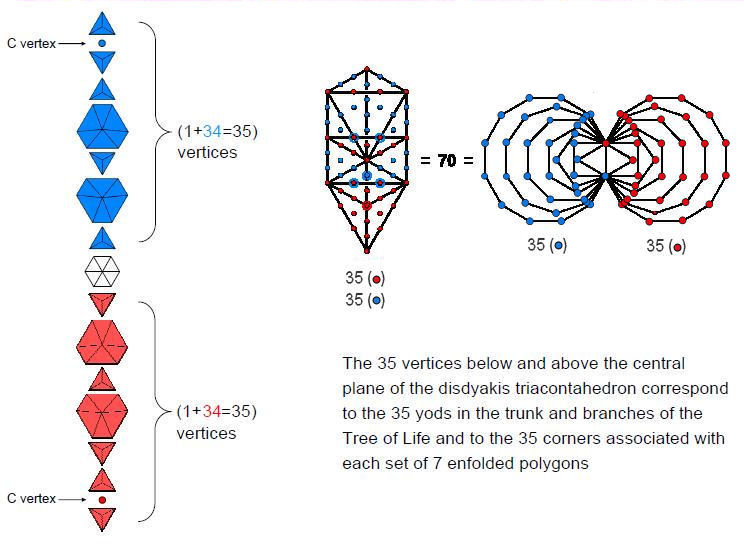Each set of seven polygons has 27 corners that are vertices of the disdyakis triacontahedron. Its centre and the 54 vertices of both sets creates a set of 55 points. This number is F10. Each set has 27 sides. Including either the six corners of the central hexagon and a C vertex on the axis or the six sides of the hexagon and the other C vertex creates two sets of 34 points & sides, where 34 is the ninth Fibonacci number F9. The number of points & lines forming the shapes of the 15 polygons = 55 + 2×34 = 123. This is the tenth Lucas number L10. The polygons constitute a geometrical realisation for n = 10 of the general relation between Lucas numbers and Fibonacci numbers: Ln = Fn + 2Fn-1 as L10 = F10 + 2F9. The factor of 2 refers to the upper and lower halves of the polyhedron, each containing seven polygons. This property is the polyhedral counterpart of the 123 geometrical elements in the 3-tree (Fig. 5, Part 1), which has 55 geometrical elements in the lowest Tree of Life and 34 elements in each of the second and third Trees. 76 polyhedral vertices & centres of the 14 polygons surround the centre of the disdyakis triacontahedron. 76 is the ninth Lucas number L9.

13There are 137 corners & sides in the set of 15 polygons and the two diametrically opposite C vertices that form the axis of the disdyakis triacontahedron. This is the single polyhedral counterpart to the 1370 yods in the inner Tree of Life (Fig. 21, Part 1) when its 94 sectors are each divided into three triangles which are then turned into tetractyses. The number 137 determining the fine-structure constant α = e2/ħc is embodied in the arrangement of the vertices of the disdyakis triacontahedron. It is determined by the tenth Lucas number L10 = 123, which is the number of corners & sides other than the centres of the 14 polygons above and below the centre of the polyhedron:   137 = 123 + 14.   It is also determined by the ninth Lucas number L9 = 76 because 76 corners/vertices surround the centre of the polyhedron:   137 = 76 + 60,   where 60 is the number of sides of the 15 polygons. 76 is the number value of YAHWEH ELOHIM, the Godname of Tiphareth.   The number 137 is embodied in the polyhedral Tree of Life as the 137 corners of the 396 internal triangles of the concentric 144 Polyhedron with 74 vertices and the disdyakis triacontahedron with 62 vertices:   137 = 1 + 74 + 62 .

14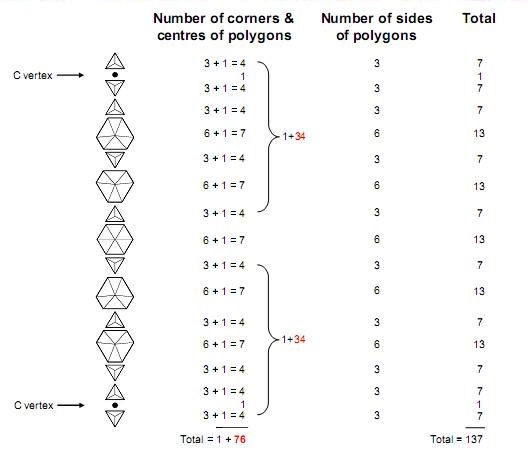The 42 triangles surrounding the central triangle in the 2-dimensional Sri Yantra have 68 corners (34 corners in each half). This leaves one other corner of the central, downward-pointing triangle and the bindu point in its middle. The 70 points creating the form of the 2-dimensional Sri Yantra are the counterpart of the 70 yods in the outer Tree of Life when its 16 triangles are tetractyses and the 70 corners of its polygonal, inner form. It demonstrates the equivalence of these sacred geometries. The two sets of 34 corners correspond to the 34 corners of the 27 sectors of each set of seven polygons (Fig. 11), whilst the bindu and lower corner of the central triangle correspond to the two C vertices on the central axis of the disdyakis triacontahedron and to the two endpoints of the root edge shared by the 14 polygons of the inner Tree of Life. Their counterpart in the outer Tree are its top (Kether) and bottom (Malkuth). More details about the analogous features of the Sri Yantra and other holistic systems are discussed in Article 35.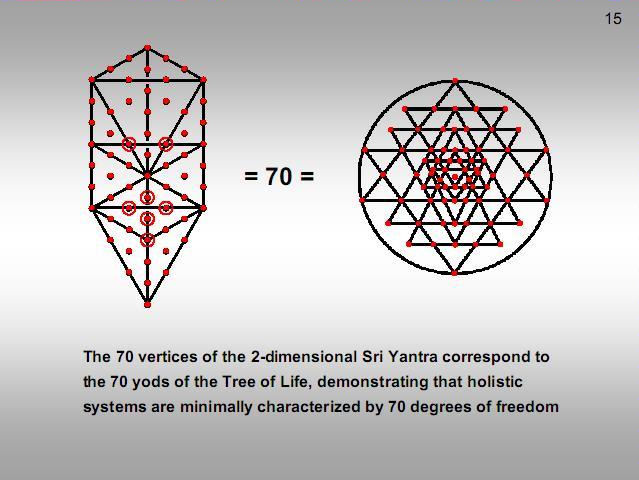The 3-dimensional Sri Yantra is also equivalent to the inner Tree of Life. As the bindu point is no longer in the plane of the central triangle, as in the 2-dimensional case, the latter constitutes a distinct geometrical element. The eight geometrical elements making up the central triangle and bindu correspond to the eight points that are either corners of the polygons that coincide with Sephiroth of the outer Tree when it is projected onto the plane containing the polygons or centres of polygons. The four sets of triangles have 252 geometrical elements. They correspond to the 252 other yods outside the root edge that make up the 47 tetractyses. YAHWEH, the Godname of Chokmah with number value 26, prescribes both the geometrical composition of the Sri Yantra and the yod population of the inner Tree of Life.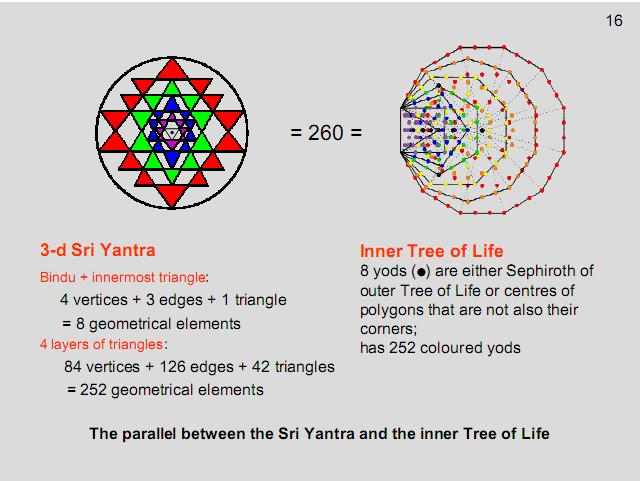The 252 geometrical elements making up the 42 triangles of the Sri Yantra that surround its central one correspond to the 252 geometrical elements composing seven overlapping Trees of Life. The seven elements in the central triangle that surround the bindu correspond to the seven elements (one point, three lines & three triangles) that are added when the seven Trees become the 7-tree.   The seven Trees of Life represent the seven planes of consciousness corresponding to the seven Sephiroth of Construction.

17Remarkable confirmation of the equivalence of seven Trees of Life and the Sri Yantra is provided by their yod populations when their triangles are tetractyses. There are 384 yods up to the top of the seventh Tree of Life in a set of n overlapping Trees (n>7) and 384 yods that belong either to the central triangle as hexagonal yods or to the 42 triangles surrounding it.The nine parent triangles that create the Sri Yantra have 27 corners. 26 of them (denoted by small circles) are corners of the 42 triangles that surround its centre. A Type B triangle is a triangle whose sectors are each divided into three sectors. It has 46 yods when the nine sectors are tetractyses. The 42 triangles has (4×42=168 ) internal corners of their (9×42=378) tetractyses. 168 is the number value of the Mundane Chakra of Malkuth. Many previous articles demonstrated that it is a structural parameter of the superstring. The 42 Type B triangles surrounding the centre of the Sri Yantra have 1680 yods other than the 26 corners that belong to the nine primary triangles and the 168 internal corners of tetractyses. This is how the Sri Yantra embodies the number 1680, recorded over a century ago by the Theosophist C.W. Leadbeater as the number of turns in each helical whorl of the particle that he claimed through his remote-viewing ability was the basic unit of matter. The sceptic may argue that the choice of yods is ad hoc and made to deliver the number 1680. This ignores the fact that the corners of tetractyses that are left out of the calculation number 168 — the very superstring structural parameter that numerous articles have proved is embodied in holistic systems. The sceptic’s assumption that this, too, occurs by chance is implausible, especially given the fact that there are 168 yods lining the sides of the 21 triangles in each half of the Sri Yantra, another occurrence of this number which he must also regard as coincidental.

19Imagine the 50 faces of the five Platonic solids divided into their 180 sectors. Then suppose that each sector is a tetractys. There are 48 hexagonal yods in the 12 tetractyses forming the four faces of the tetrahedron, 96 hexagonal yods in the eight faces of the octahedron, 96 hexagonal yods in the six faces of the cube, 240 hexagonal yods in the 12 faces of the icosahedron & 240 hexagonal yods in the 12 faces of the dodecahedron. 48 is the number value of Kokab , the Mundane Chakra of Malkuth. The four Platonic solids associated by the ancient Greeks with the four Elements of Earth, Water, Air & Fire have 480 hexagonal yods (240 hexagonal yods in the tetrahedron, octahedron & cube and 240 hexagonal yods in the icosahedron).

20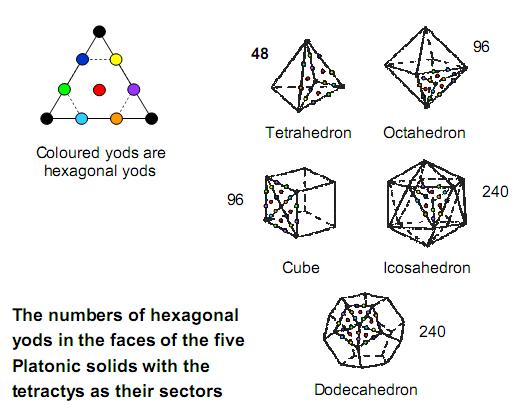The counterpart of this property in the outer Tree of Life are the 240 yods added to the lowest Tree when the sectors of its 19 triangles are turned into tetractyses. Its counterpart in the inner form of the Tree of Life are the 240 hexagonal yods that are added to each set of seven separate polygons when their sectors are turned into tetractyses. Each hexagonal yod symbolizes one of the 240 roots of the rank-8, exceptional Lie group E8, the (240+240=480) hexagonal yods in the two sets denoting the 480 roots of E8×E8, which is the symmetry group of the unified interaction of one of the two types of heterotic superstring.

21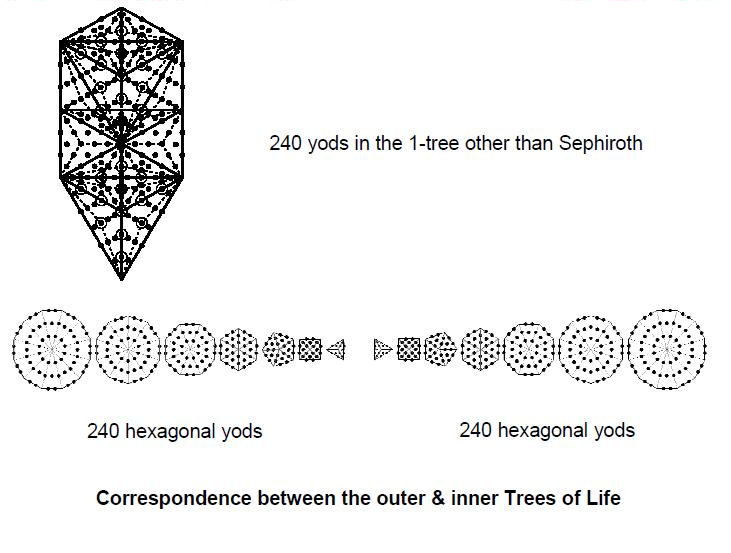The 62 vertices of the disdyakis triacontahedron are the vertices of 28 Platonic & Catalan solids. 21 polyhedra are copies of the first four Platonic solids. The seven other polyhedra that can be fitted into the disdyakis triacontahedron comprise the dodecahedron, five rhombic dodecahedra and one rhombic triacontahedron.Suppose that the faces of the 28 Platonic & Catalan solids formed by vertices of the disdyakis triacontahedron are constructed from tetractyses. There are 1680 hexagonal yods in the faces of the 21 copies of the first four Platonic solids and 1680 hexagonal yods in the faces of the other seven polyhedra. The 28 polyhedra therefore have 3360 hexagonal yodsThe number 3360 is embodied in the inner Tree of Life as the 3360 yods in its seven enfolded polygons when their 47 sectors are each turned into the so-called ‘2nd-order tetractys.’ 47 is the eight Lucas number L8. This is generated from the Pythagorean tetractys (the 1st-order tetractys) by regarding each of its ten points as a tetractys. This higher-order tetractys has 85 yods, where 85 = 40 + 41 + 42 + 43. 84 yods surround its centre, where  84 = 12 + 32 + 52 + 72. As a parameter of all holistic systems, the number 3360 has the following significance for the structure of the superstring: each of the ten whorls of the unit of matter described by the Theosophists Annie Besant & C.W. Leadbeater is a helix with 1680 turns. It twists five times around the spin axis of the particle, so that it winds (1680/5=336) times in one revolution. The ten whorls wind 3360 times in one revolution and 1680 times in a half-revolution. The 21 copies of the first four Platonic solids and the seven other polyhedra embody the number of circularly polarised oscillations in the ten whorls as they make these two half-revolutions.The numbers of Platonic solids of the first four types that can be fitted into the disdyakis triacontahedron and which have 1680 hexagonal yods in their faces are the letter values of EYHEH (“I am”), the Godname of Kether, whose number value is 21. It is also the eighth Fibonacci number F8. This one of the ways in which this Godname prescribes the superstring structural parameter 1680.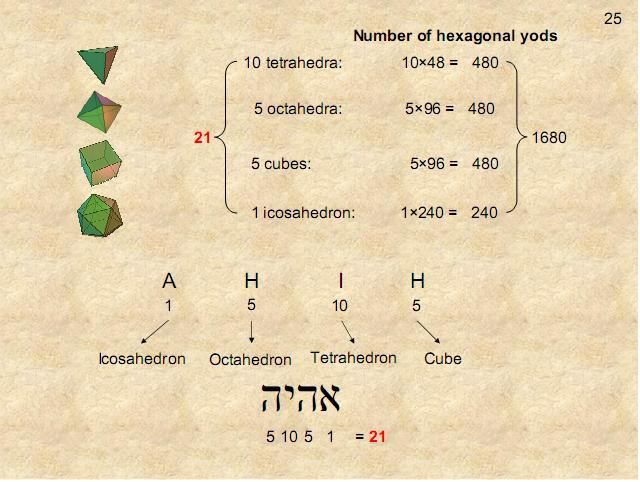The number 168 is the number value of Cholem Yesodeth, the Mundane Chakra of Malkuth. Confirmation that it refers to the Malkuth aspect of the Tree of Life is the remarkable fact that 49 overlapping Trees of Life have 1680 corners, sides & triangles. The lowest 49 Trees in CTOL map the 49 subplanes of the cosmic physical plane. Each cosmic plane corresponds to a Sephirah of Construction, and the cosmic physical plane corresponds to Malkuth, the physical level of CTOL in a cosmic sense. 49 is the number value of EL CHAI, the Godname of Yesod.

26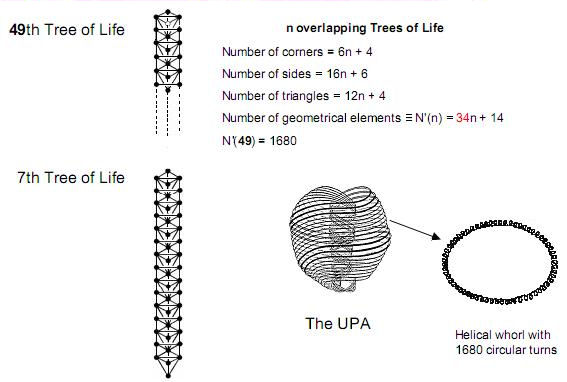Joining the 62 vertices of the disdyakis triacontahedron to its centre creates 180 triangles in its interior with (180×3=540) sectors. There are 60 vertices surrounding an axis drawn through any two opposite vertices. The sectors of the internal triangles have 180 internal corners and (60 + 3×180 = 600) internal sides surrounding the centre of the polyhedron. Its 120 faces are 120 triangles with 180 sides and 60 corners surrounding the axis. 1680 corners/vertices, sides/edges & triangles surround this axis. The disdyakis triacontahedron embodies the superstring structural parameter 1680. Its 900 triangles have 780 corners & sides.

27The 1680 geometrical elements that surround any axis of the disdyakis triacontahedron is the polyhedral realisation of the number value 168 of Cholem Yesodeth, the Mundane Chakra of Malkuth. That this is no coincidence is indicated by the fact that the 780:900 division of geometrical elements reflects, apart from the Tree of Life/tetractys factor of 10, the gematria number values of both Cholem (78) and Yesodeth (90). In accordance with the rules of gematria, the letter value 400 of tav, the final Hebrew letter in Yesodeth , can be contracted to 4.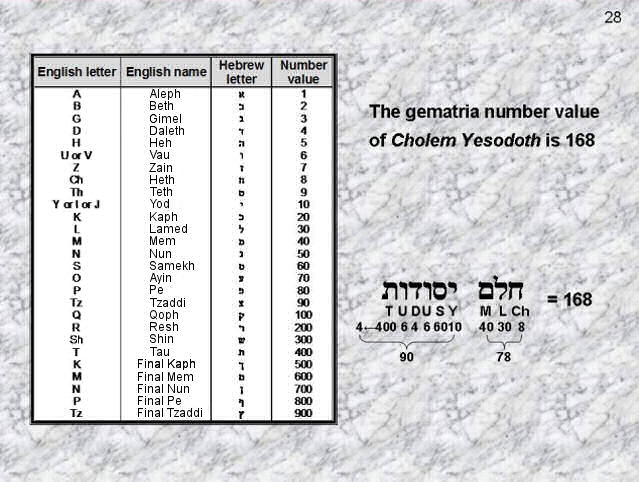The disdyakis triacontahedron is unique in that no other Archimedean or Catalan solid has 1680 geometrical elements surrounding an axis that passes through two opposite vertices. This is true for polyhedra with triangular faces whether their faces are regarded as single triangles (case A) or whether they are divided into their sectors (case B). It is also true for polyhedra having faces that are not triangular.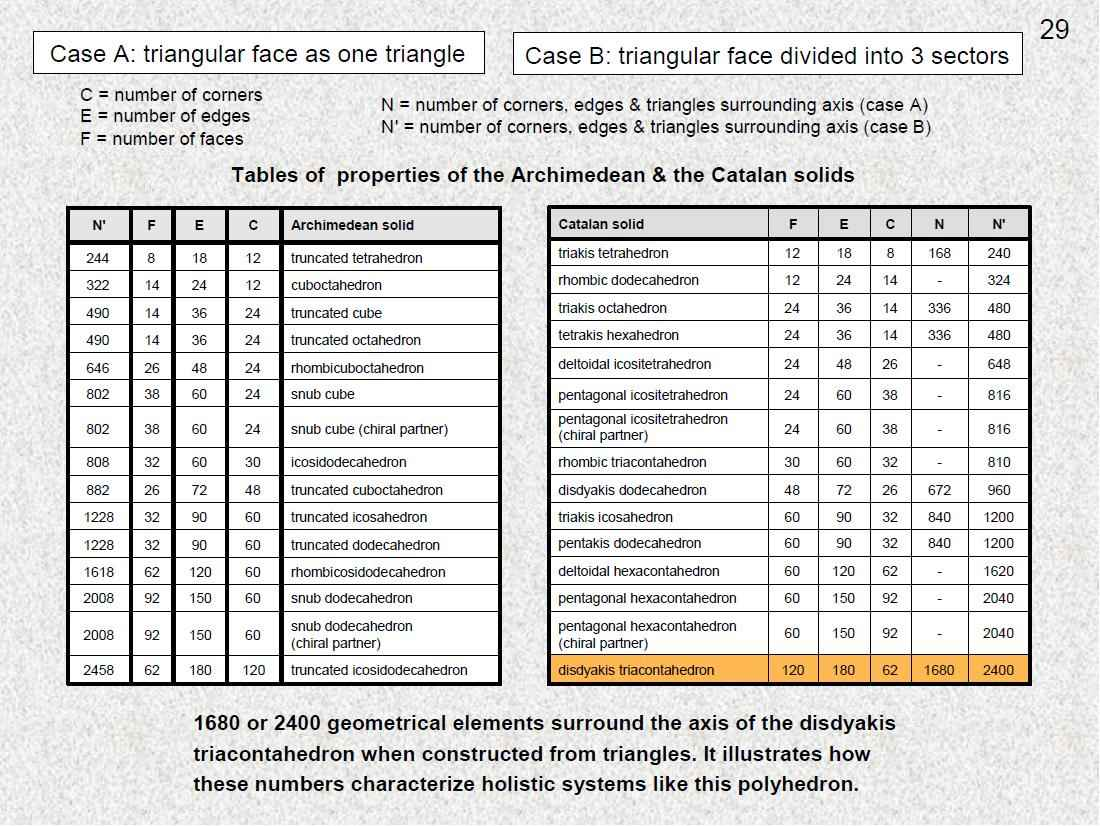ADONAI, the Godname of Malkuth (the physical universe), has number value 65 . It prescribes the ten lowest Trees of Life in CTOL because they have 65 SLs. These Trees map the ten spatial dimensions predicted by M-theory, the general theory being currently being sought by physicists to explain the basic properties of physical matter and the forces of nature. A decagon within a square is a representation of the ten Trees because the object has 65 yods when the ten sectors of the decagon are tetractyses. The four corners of the square correspond to Kether, Chokmah, Binah & Daath of the tenth Tree and the 61 yods in the decagon correspond to the 61 SLs up to Chesed of this Tree. The letter values of ADONAI are the numbers of different yods.

30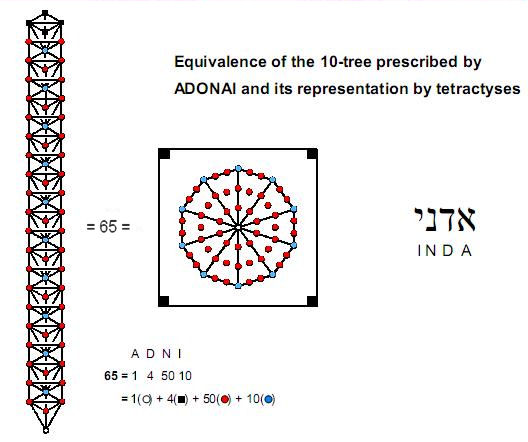When the triangles in the 10-tree prescribed by ADONAI are divided into their sectors and each sector turned into a tetractys, there are 1680 yods below the apex of the tenth Tree of Life (65th SL), including those outside it that belong to the eleventh Tree. Each yod denotes one of the circular turns in a whorl of the heterotic superstring described by the Theosophists Besant & Leadbeater with the aid of a form of remote-viewing known in yoga as one of the siddhis, or paranormal abilities. The same number has been encountered in this article as: the 1680 yods in the 42 so-called ‘Type B’ triangles of the Sri Yantra other than internal corners of tetractyses and the corners of its nine parent triangles; the 1680 points, lines & triangles making up the disdyakis triacontahedron that surround an axis passing through any two diametrically opposite vertices;  the 1680 hexagonal yods in the faces of the five Platonic solids, the rhombic triacontahedron & the disdyakis triacontahedron;  the 1680 hexagonal yods in the faces of the 21  copies of the first four Platonic solids whose vertices belong to the disdyakis triacontahedron.  The n-tree has (12n+7) triangles with (16n+9) sides. The number of sides in their (36n + 21) sectors is 16n + 9 + 3(12n+7) = 52n + 30.  The 10-tree has 361 sectors with 550 sides. This is the counterpart of the 550 geometrical elements in the 50 faces of the five Platonic solids, the 550 Sephiroth in CTOL and the 550 unshared geometrical elements in the 15 polygons of the disdyakis triacontahedron. The tenth Fibonacci number 55 determines the form of all such holistic systems. In particular, it determines the form of each of the ten helices that comprise the E8×E8 heterotic superstring.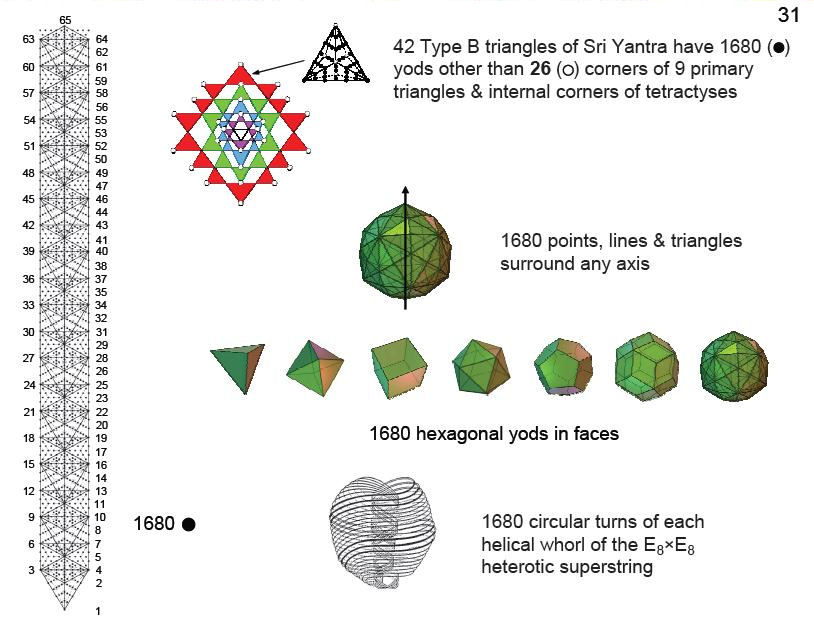Tree levels are defined as the formative stages in the Fibonacci number-governed growth of successive Trees of Life in CTOL. The number of tree levels in the n-tree is:   t(n) = 3n + 4.   t(1) = 7, which is the fourth Lucas number, t(3) = 13, which is the seventh Fibonacci number and t(10) = 34, which is the ninth Fibonacci number. This number has appeared in this article:   1. in the outer Tree of Life as the number of geometrical elements in the Upper Face of the 1-tree and as the number of elements added by successive Trees; 2. in the inner Tree of Life as the 34 corners associated with each set of seven enfolded polygons that are intrinsic to them because they do not coincide with those of polygons enfolded in the next higher Tree; 3. as the 34 single bones of the axial skeleton of the human body; 4. as the 34 vertices & centres of the first four Platonic solids; 5. in the disdyakis triacontahedron as the 34 corners of the 27 sectors of the seven polygons either above or below the central hexagon that are formed by vertices of this polyhedron.   Remarkably, it determines the very number of overlapping Trees of Life that have a yod population of 1680. In this way, it determines the very helical structure of each whorl of the heterotic superstring. The sum 34 = 21 + 13 differentiates between large-scale, 3-dimensional space, which is mapped by the 3-tree with 13 tree levels, and the next 21 tree levels that span seven Trees up to the 34th, which marks the top of the 10-tree mapping the ten spatial dimensions. In other words, the eighth Fibonacci number 21 measures the seven compactified dimensions predicted by M-theory. The tenth Fibonacci number 55 determines the 10-tree (and therefore the superstring structural parameter 1680) because their 127 triangles have 361 sectors with 550 (=55 ×10) sides.

32References Phillips, Stephen M. Article 27: “How the disdyakis triacontahedron embodies the structural parameter 1680 of the E8×E8 heterotic superstring,” (WEB, PDF). Besant, A. & Leadbeater, C.W. “Occult Chemistry,” 3rd ed., Theosophical Publishing House, Adyar, Madras, India, 1951. Phillips, Stephen M. Article 36: “The Sri Yantra-like pattern of the 15 layers of vertices in the disdyakis triacontahedron and its scientific meaning,” (WEB, PDF). Phillips, Stephen M. Article 49: “How some sacred geometries are equivalent maps of all levels of reality,” (WEB, PDF). Phillips, Stephen M. Article 35: “The Tree of Life nature of the Sri Yantra and some of its scientific meanings,” (WEB, PDF).

Home